# 如何合并多个 Excel 表格文件至一个总表格

1. 把需要合并的多个 Excel 表格放在同一文件夹内，并在该文件夹下新建一个 Excel 文件。（图中以 Merge.xlsx 举例）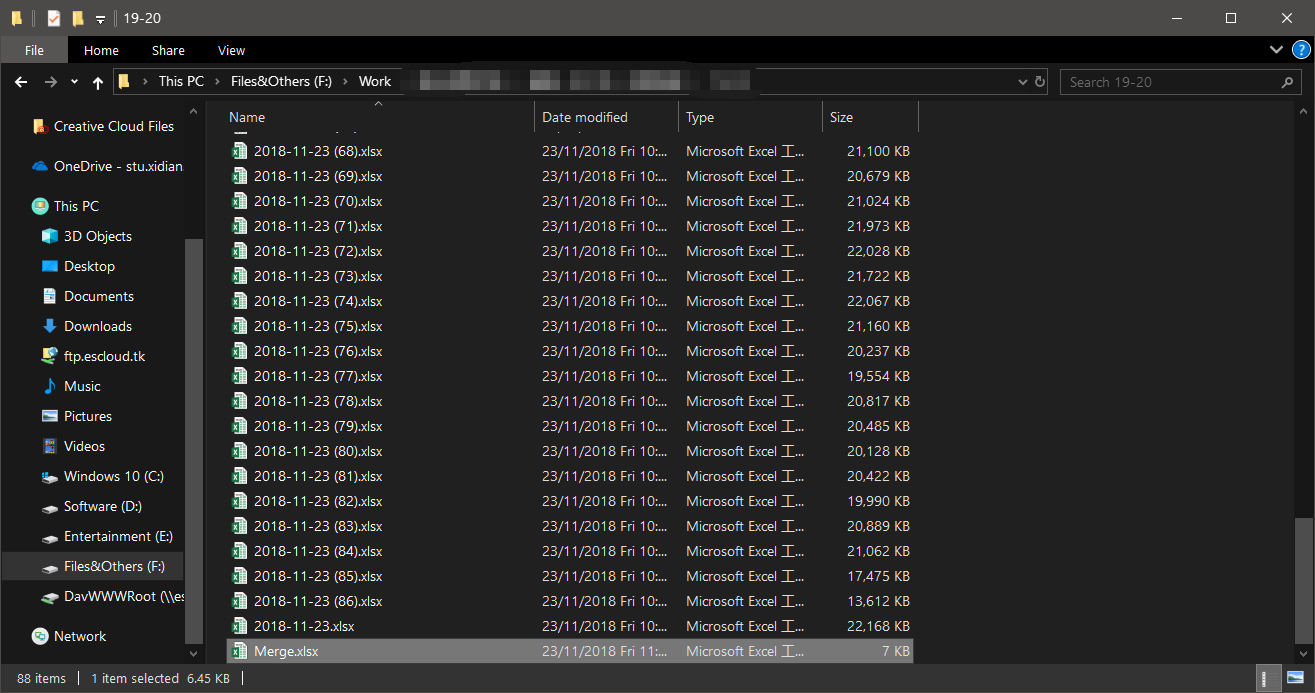2. Microsoft Excel 打开刚刚新建的 Excel 文件 Merge.xlsx ，在表格下方右键单击 `sheet1` ，选择 `查看代码` 。点击后进入宏计算界面。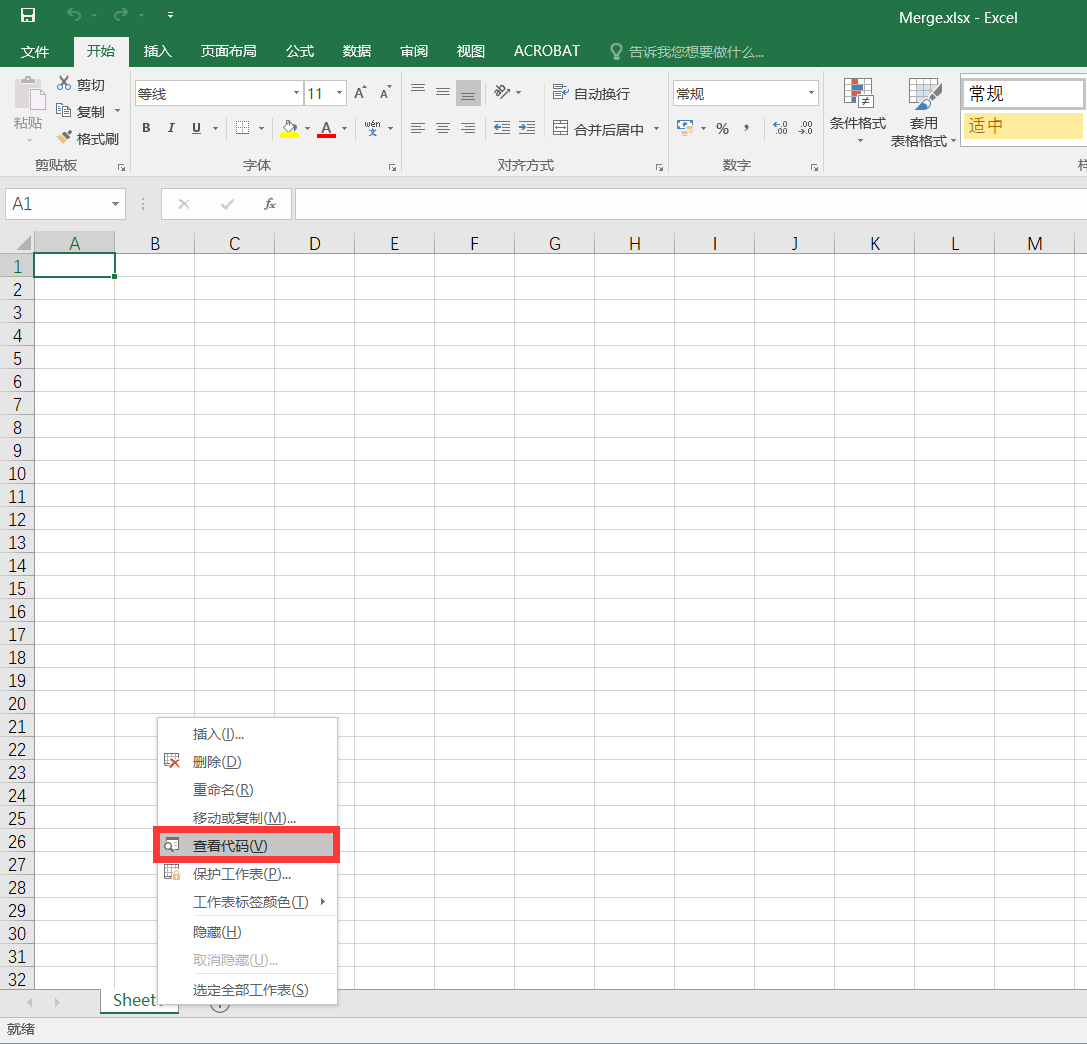3. 在代码窗口中输入以下代码：

``````Sub 合并当前目录下所有工作簿的全部工作表()
Dim MyPath, MyName, AWbName
Dim Wb As Workbook, WbN As String
Dim G As Long
Dim Num As Long
Dim BOX As String
Application.ScreenUpdating = False
MyPath = ActiveWorkbook.Path
MyName = Dir(MyPath & "\" & "*.xls")
AWbName = ActiveWorkbook.Name
Num = 0
Do While MyName <> ""
If MyName <> AWbName Then
Set Wb = Workbooks.Open(MyPath & "\" & MyName)
Num = Num + 1
With Workbooks(1).ActiveSheet
.Cells(.Range("B65536").End(xlUp).Row + 2, 1) = Left(MyName, Len(MyName) - 4)
For G = 1 To Sheets.Count
Wb.Sheets(G).UsedRange.Copy .Cells(.Range("B65536").End(xlUp).Row + 1, 1)
Next
WbN = WbN & Chr(13) & Wb.Name
Wb.Close False
End With
End If
MyName = Dir
Loop
Range("B1").Select
Application.ScreenUpdating = True
MsgBox "共合并了" & Num & "个工作薄下的全部工作表。如下：" & Chr(13) & WbN, vbInformation, "提示"
End Sub
``````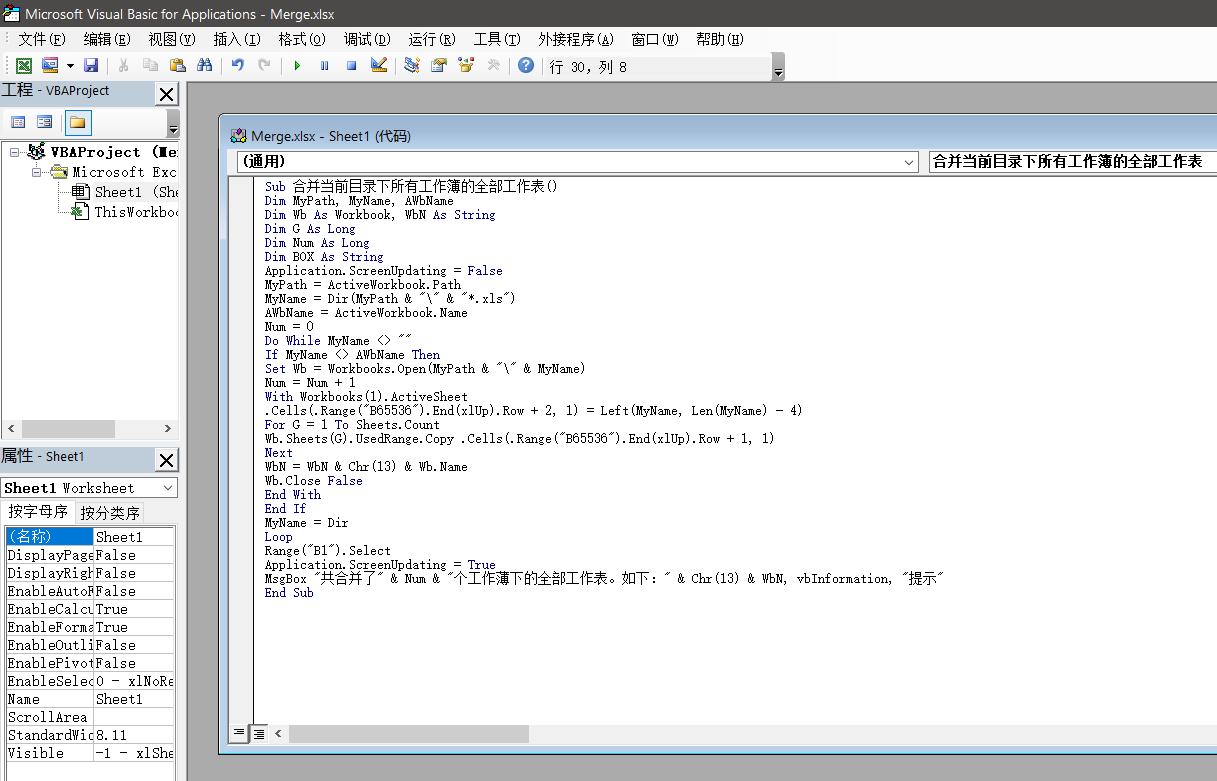4. 在上方菜单栏点击 `运行 - 运行子过程/用户窗体`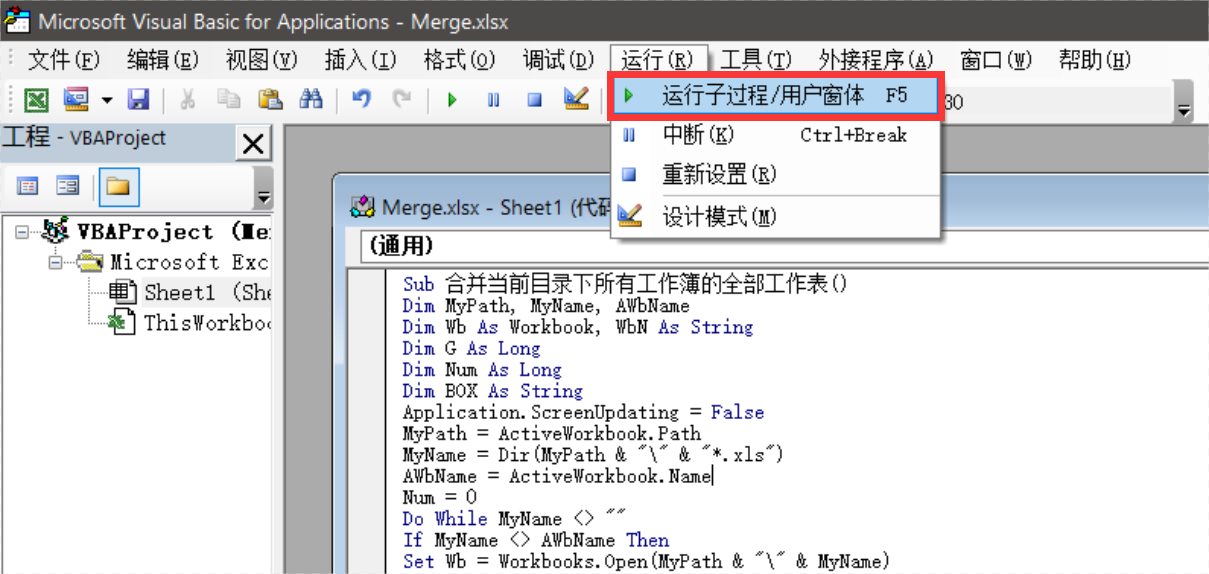5. 点击 `运行` 之后稍作等待，等待时间大部分取决于表格数量。运行完毕后会提示已合并 x 个文件，点击确定即可查看、保存合并后的表格，该表格包括同文件夹下所有表格文件的内容。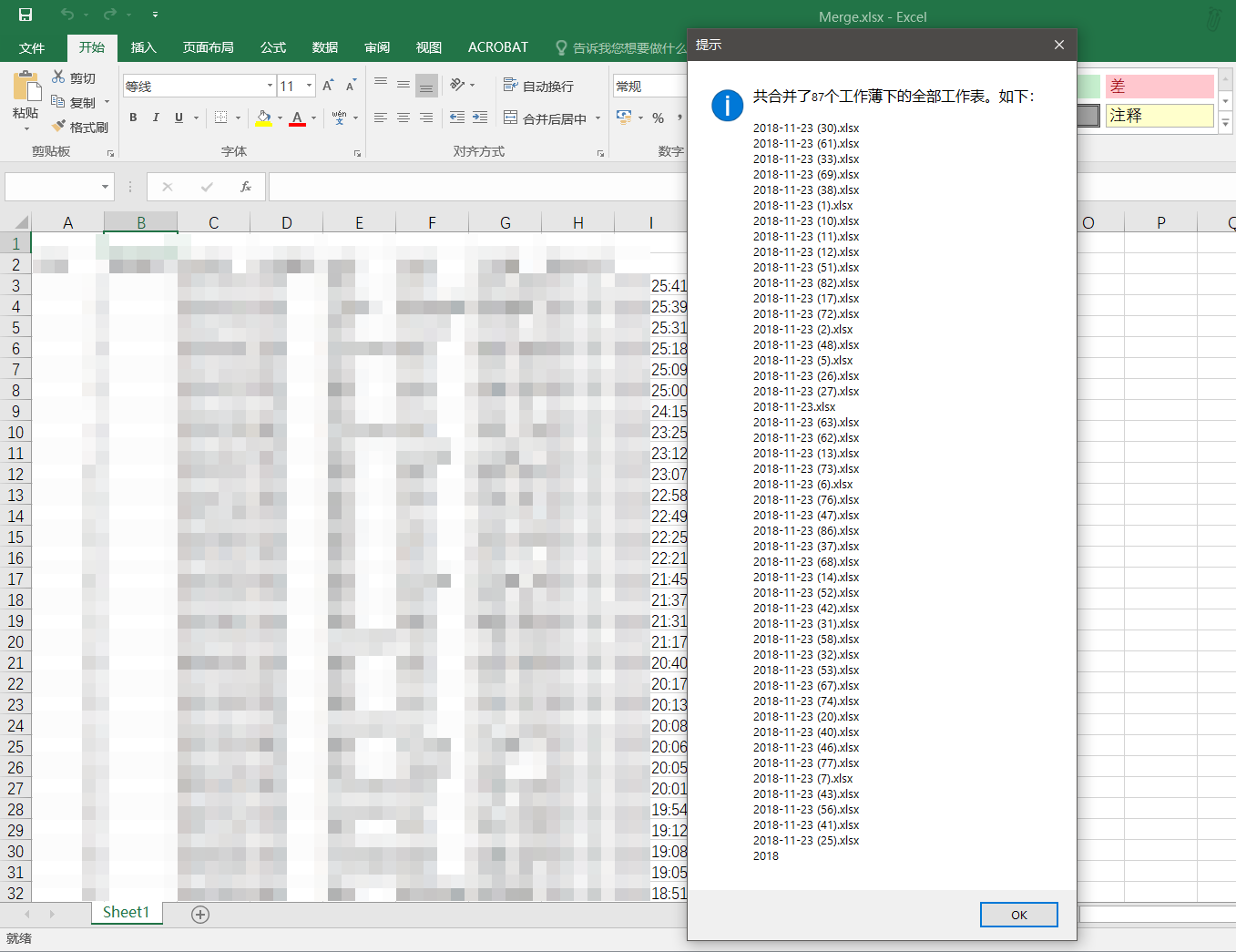### One Comment

1. Pingback: viagra